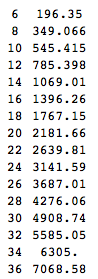Jump to content

# Help, I'm a dummy...

## Recommended Posts

Ok, I want to build a small boat which will consist basically of a floating platform supported by 2 pontoons which are 8 feet in length. What diameter do the pontoons have to be to support a total weight (platform plus load) of 700 lbs.? Any help would be appreciated, especially if someone could provide an easily understood formula or explain in simple terms how they arrived at the answer. I'm really not THAT dumb but it's been a long time since high school...##### Share on other sites

So let's suppose the two pontoons are perfect cylinders. The force of buoyancy is

$F_B=\rho V$

where [imath]\rho[/imath] is the density of water and V the volume displaced by the pontoons. (For those of you about to complain about the missing g: I'll use a density in pounds, giving me a buoyant force in pounds.) Now, the volume of a cylinder is

$V = \pi r^2 h = \frac \pi 4 d^2 h$

where r is its radius and h the length. Since you want diameter, I converted that to be in terms of d, the diameter.

Let's put that together and multiply by two, for two pontoons:

$F_B = \frac \pi 2 \rho d^2 h$

Now, some numbers:

$\rho = 62.5 \; \frac{\text{pounds}}{\text{foot}^3}$

$h = 8 \text{ feet}$

So, for example, suppose your pontoons are two feet in diameter. The maximum buoyant force they can provide is

$F_B \approx 3140 \text{ pounds}$

On the other hand, if you know the boat weight and you just need to know pontoon diameter, just solve for d:

$d = \sqrt{\frac{2 F_B}{\pi \rho h}}$

You said you need to support 700 pounds; add in the weight of the pontoons themselves, plus a fat margin of error (you don't want the pontoons to be fully submerged underwater, after all) and say you want a maximum load of 3000 pounds, and you can plug in the numbers and get close to two feet.

##### Share on other sites

For safety

Is this calculation wrong?

FB =~ 5 x total load + platform weight + two pontoons weight

FB =~ 4 x total load +700+ two pontoons weight

Edited by alpha2cen
##### Share on other sites

ok, the math is still a little deep for an old-timer, so let me put the question another way; how much would my 2 pontoons support if they were 8 feet long and 6 inches in diameter? How about 8 inches? And 10?

##### Share on other sites

Two six-inch diameter pontoons could support about 200 pounds; eight-inch pontoons would handle 350 pounds, and ten-inch pontoons 550.

Here, have a table. On the left is diameter in inches, and on the right is how much payload could be carried by two eight-foot pontoons with that diameter:Be sure to give yourself extra capacity -- this is the maximum payload with the pontoons fully submerged, and you probably don't want that.

##### Share on other sites

Be sure to give yourself extra capacity -- this is the maximum payload with the pontoons fully submerged, and you probably don't want that.

To maintain reserve buoyancy, don't submerge the pontoons beyond the halfway point, otherwise the boat can capsize more easily.

##### Share on other sites

Excellent table C'pn, thanks! And thanks for all responses...

## Create an account or sign in to comment

You need to be a member in order to leave a comment

## Create an account

Sign up for a new account in our community. It's easy!

Register a new account

## Sign in

Already have an account? Sign in here.

Sign In Now
×

• #### Activity

• Leaderboard
×
• Create New...

## Important Information

We have placed cookies on your device to help make this website better. You can adjust your cookie settings, otherwise we'll assume you're okay to continue.